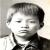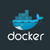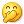## 最近写书的部分内容 热

typedef struct {
//_i64 pheader;
_p hp;//header base p;
_I s;//size
_I t;//tail not used
_I h;//head
_I l;//len ,avail data num
_u8 p;//have bug if used such as BUS_S a;;so not allow used array mode
}_D_BUF;
typedef _D_BUF * _P;

#define _SIZE(Q) (Q)->s
#define _LEN(Q) (Q)->l
#define _HEAD(Q) (Q)->h
#define _TAIL(Q) (Q)->t
#define ___offset(m,_T_)  ((_I)(_I64)&((_T_*)0)->m)  //not support 64 index
#define _mp2SP(pv,_T_) ((_T_ *)((_p)(pv) - ___offset(p,_T_)))

#define _Pheader(P,_T_) ((_T_*)((P)->hp))
#define _Pp(P) ((P)->p)
#define _pP(pv) _mp2SP(pv,_D_BUF)
#define _ph(p) _HEAD(_pP(p))
#define _ps(p) _SIZE(_pP(p))
#define _pt(p) _TAIL(_pP(p))
#define _pl(p) _LEN(_pP(p))
#define _pheader(p,_T_) ((_T_*)(_pP(p)->hp))

＝＝＝＝＝＝下面是正文摘抄＝＝＝＝＝＝＝＝

#define __empty_unit(pu)

#define __cp_unit(pu,dst,src)

#define _DISCARD_UNIT(_NAME,_T_) static _I discard_##_NAME(_p p){\

_T_ *phead = (_T_ *)p;\

_T_ *ptail = (_T_ *)(p + _pl(p));\

_I w,r;\

if (phead == ptail){\

return 0;\

}\

r = 0;\

while ((!__empty_unit(phead + r))&&(phead + r < ptail)){\

r++;\

}\

w = r;\

while (phead +r < ptail){\

if (__empty_unit(phead + r)){\

r++ ;continue;\

}\

__cp_unit(phead,w,r);\

w++;\

r++;\

}\

_pl(p) -= (r - w) * sizeof(_T_);\

return w;\

}

#undef __empty_unit

#undef __cp_unit

#define __empty_unit(pu) (*(pu) == 0)

#define __cp_unit(pu,dst,src) do {*(pu + dst) = *(pu + src);}while (0)

_DISCARD_UNIT(_i32,_i32)

#undef __empty_unit

#undef __cp_unit

#define __empty_unit(pu) (*(pu) == 0)

#define __cp_unit(pu,dst,src) do {*(pu + dst) = *(pu + src);}while (0)

_DISCARD_UNIT(_i16,_i16)

_i32 main(_i32 argc,_s argv[]){

init_m_alloc(0,0);

_I i;

_P P = C_MALLOC(sizeof(_i32)*100,0);

_p p= _Pp(P);

_i32 * tmp = (_i32*)p;

for (i = 0 ;i < 100 ; i++){

tmp[i] = i;

}

for (i = 0 ;i < 100 ; i++){

if (tmp[i] & 0x7){

}else{

tmp[i] = 0;

}

}

_pl(p) = sizeof(_i32) * 100;

_sa(p,_pl(p));

discard__i32(p);

_sa(p,_pl(p));

discard__i16(p);

_sa(p,_pl(p));

C_FREE(P);

return 0;

}

#undef __empty_unit

#undef __cp_unit

#define __empty_unit(pu) (*(pu) == 0)

#define __cp_unit(pu,dst,src) do {*(pu + dst) = *(pu + src);}while (0)

_DISCARD_UNIT(_i32,_i32)

static discard__i32(_p p) ....的函数。

======= 结束＝＝＝＝＝＝＝

#define _IS_EMPTY_OP(pof) (((pof)->m ==0) && ((pof)->n== 0))

#define _CP_OP(pof,dst,src) do {\
(pof+dst)->a = (pof+src)->a;\
(pof+dst)->n = (pof+src)->n;\
(pof+dst)->b = (pof+src)->b;\
(pof+dst)->m = (pof+src)->m;\
(pof+dst)->len = (pof+src)->len;\
(pof+dst)->next = (pof+src)->next;\
}while (0)

#undef __empty_unit
#undef __cp_unit
#define __empty_unit(pu) _IS_EMPTY_OP(pu)
#define __cp_unit(pu,dst,src) _CP_OP(pu,dst,src)
_DISCARD_UNIT(op_info,_OP_FILE_S)

0#### 引用来自“看能不能改个名”的答案

0#### 引用来自“ueharaai”的答案

free有什么好痛恨的。好借好还，再借不难。痛恨free，怕是占有欲太强了。哈。
0#### 引用来自“享开发”的答案

0路过，捧个场